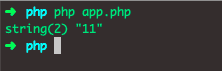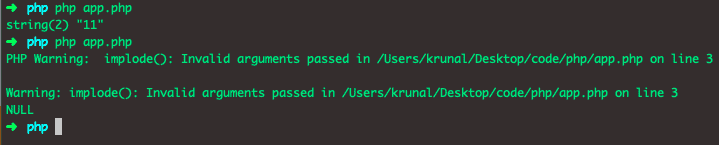# PHP Implode Example | PHP implode() Function TutorialPHP Implode Example | PHP implode() Function Tutorial is today’s topic. The implode function in PHP is used to join the elements of an array with a string. The implode() function returns the string from the elements of the array. The function is binary-safe. The implode() function returns a string containing the string representation of all the array items in the same order, with a glue string between each element. We join the array elements with the string. Just like join() function, implode() function also returns the string formed from the elements of the array.

## PHP Implode Example

If we have an array of elements, we can use the implode() function to join them all to form one string. See the syntax of PHP implode() function.

### Syntax

`implode(separator,array)`

The separator parameter is optional and specifies what to put between the array elements.

The array parameter is required, and it is the array to join to the string.

See the following example.

```<?php

// app.php

\$frontend = ['Svelete','Angular','React', 'Vue'];
echo implode("|", \$frontend);```

See the below output.The implode() function accept its parameters in either order. However, for consistency with the explode() function, you should use the documented order of arguments.

One thing you can note that an array with one or no elements works fine. For example, see the code.

```<?php

// app.php

\$arrA = ["Asylum","House","Coven"];
\$arrB = ["AHS"];
\$arrC = [];

echo implode("|", \$arrA)."\n";
echo implode("|", \$arrB)."\n";
echo implode("|", \$arrC);```

See the below output.The implode() function in PHP is easily remembered as “array to a string,” which means that it takes an array and returns a string. It rejoins any array elements and returns the resulting string, which may be put in a variable.

## PHP implode() function on Associative Arrays

The implode function acts on array “values,” disregarding any keys. See the following example.

```<?php

// app.php

\$devs = ['1st' => 'CloudArchitecht',
'2nd' => 'DevOps',
'3rd' => 'DataScientists'];

echo implode(" | ", \$devs)."\n";
```

See the below output.The implode() function also be used for building tags or complex lists, like the following.

```<?php

\$elements = array('a', 'b', 'c');

echo "<ul><li>" . implode("</li><li>", \$elements) . "</li></ul>";```

Also, it is quite handy in INSERT statements.

```<?php

// array containing data
\$array = array(
"name" => "Krunal",
"surname" => "Lathiya",
"email" => "krunal@appdividend.com"
);

// build query...
\$sql  = "INSERT INTO table";

// implode keys of \$array...
\$sql .= " (`".implode("`, `", array_keys(\$array))."`)";

// implode values of \$array...
\$sql .= " VALUES ('".implode("', '", \$array)."') ";

// execute query...
\$result = mysql_query(\$sql) or die(mysql_error());
```

If you want to implode the array of booleans, you will get strange result. See the following code.

```<?php

// app.php

var_dump(implode('', array(false, true, false, false, true)));```

The output is following.It is worth noting that if you call implode on the string rather than the array, you do not get your string back, you get NULL.The null values are imploded too. You can use an array_filter() to sort out the null values.

```<?php

\$ar = array("krunal", null, "lathiya");

print(implode(',', array_filter(\$ar, function(\$v){ return \$v !== null; })));```

Finally, PHP Implode Example | PHP implode() Function Tutorial is over.

The post PHP Implode Example | PHP implode() Function Tutorial appeared first on AppDividend.